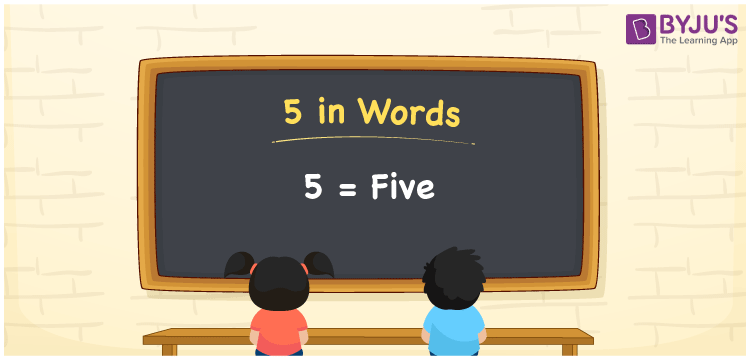# 5 in Words

5 in words can be written as Five. In this article 5 in words, the concept of counting is explained in the simplest way possible by keeping in mind the understanding abilities of the students. If you spend Rs. 5 on buying a chocolate, then you can say that “I bought a chocolate for five rupees”. The conversion of 5 into words is discussed here in brief. We can write numbers in words with the help of English Alphabets. Therefore, you can read 5 as “Five” in English.

 5 in words Five Five in Numbers 5

## 5 in English Words## How to Write 5 in Words?

The concept of writing 5 in words is discussed here. One digit is present in the 5. The place value chart for the one digit of the number 5 can be understood using the table.

 Ones 5

The number 5 using expanded form can be written as:

5 × One

= 5 × 1

= 5

= Five

Therefore, 5 in words is written as Five.

5 is a natural number that precedes 6 and succeeds 4.

5 in words – Five

Is 5 an odd number? – Yes

Is 5 an even number? – No

Is 5 a perfect square number? – No

Is 5 a perfect cube number? – No

Is 5 a prime number? – Yes

Is 5 a composite number? – No

## Frequently Asked Questions on 5 in Words

Q1

### How can the number 5 be written in words?

5 can be written in words as “Five”.
Q2

### Is 5 an odd number?

Yes, 5 is an odd number as it is not divisible by 2.
Q3

### How can we write Five in numbers?

Five can be written in numbers as 5.